# Homework 1. Exploratory Data Analysis¶

Due: Thursday, September 18, 2014 11:59 PM

Download this assignment

## Introduction¶

In this homework we ask you three questions that we expect you to answer using data. For each question we ask you to complete a series of tasks that should help guide you through the data analysis. Complete these tasks and then write a short (100 words or less) answer to the question.

#### Data¶

For this assignment we will use two databases:

1. The Sean Lahman's Baseball Database which contains the "complete batting and pitching statistics from 1871 to 2013, plus fielding statistics, standings, team stats, managerial records, post-season data, and more. For more details on the latest release, please read the documentation."

2. Gapminder is a great resource that contains over 500 data sets related to world indicators such as income, GDP and life expectancy.

#### Purpose¶

In this assignment, you will learn how to:

a. Load in CSV files from the web.

b. Create functions in python.

C. Create plots and summary statistics for exploratory data analysis such as histograms, boxplots and scatter plots.

#### Useful libraries for this assignment¶

In :
# special IPython command to prepare the notebook for matplotlib
%matplotlib inline

import numpy as np
import pandas as pd
import matplotlib.pyplot as plt

# For this assignment, we need to load in the following modules
import requests
import StringIO
import zipfile
import scipy.stats


## Problem 1¶

In Lecture 1, we showed a plot that provided evidence that the 2002 and 2003 Oakland A's, a team that used data science, had a competitive advantage. Since, others teams have started using data science as well. Use exploratory data analysis to determine if the competitive advantage has since disappeared.

#### Problem 1(a)¶

Load in these CSV files from the Sean Lahman's Baseball Database. For this assignment, we will use the 'Salaries.csv' and 'Teams.csv' tables. Read these tables into a pandas DataFrame and show the head of each table.

Hint Use the requests, StringIO and zipfile modules to get from the web.

In :
#your code here

def getZIP(zipFileName):
r = requests.get(zipFileName).content
s = StringIO.StringIO(r)
zf = zipfile.ZipFile(s, 'r') # Read in a list of zipped files
return zf


Here, we use the requests, StringIO and zipfile modules to extract all the text files from the web. The zipfile model can create, read, write, append, and list ZIP files. You did not have to create a function, but I did to make the solution cleaner.

Using the URL linking to the .zip file, we can print all the files listed in the zipped folder.

In :
url = 'http://seanlahman.com/files/database/lahman-csv_2014-02-14.zip'
zf = getZIP(url)
print zf.namelist()

['SchoolsPlayers.csv', 'SeriesPost.csv', 'Teams.csv', 'TeamsFranchises.csv', 'TeamsHalf.csv', 'AllstarFull.csv', 'Appearances.csv', 'AwardsManagers.csv', 'AwardsPlayers.csv', 'AwardsShareManagers.csv', 'AwardsSharePlayers.csv', 'Batting.csv', 'BattingPost.csv', 'Fielding.csv', 'FieldingOF.csv', 'FieldingPost.csv', 'HallOfFame.csv', 'Managers.csv', 'ManagersHalf.csv', 'Master.csv', 'Pitching.csv', 'PitchingPost.csv', 'readme2013.txt', 'Salaries.csv', 'Schools.csv']

In :
tablenames = zf.namelist()
tablenames[tablenames.index('Salaries.csv')]

Out:
'Salaries.csv'

Next, we extract the 'Salaries.csv' file from the zipped folder. We use the zf.open() function to open a specific file and use pd.read_csv() to read the table into a pandas DataFrame. This table contains salaries labled by year, by player, by league and by team.

In :
salaries = pd.read_csv(zf.open(tablenames[tablenames.index('Salaries.csv')]))
print "Number of rows: %i" % salaries.shape
salaries.head()

Number of rows: 23956

Out:
yearID teamID lgID playerID salary
0 1985 BAL AL murraed02 1472819
1 1985 BAL AL lynnfr01 1090000
2 1985 BAL AL ripkeca01 800000
3 1985 BAL AL lacyle01 725000
4 1985 BAL AL flanami01 641667

Finall, we extract the 'Teams.csv' file from the zipped folder. This table contains a large amount of information, but for our purposes, we are interested in the yearID, teamID and number of wins W.

In :
teams = pd.read_csv(zf.open(tablenames[tablenames.index('Teams.csv')]))
teams = teams[['yearID', 'teamID', 'W']]
print "Number of rows: %i" % teams.shape
teams.head()

Number of rows: 2745

Out:
yearID teamID W
0 1871 PH1 21
1 1871 CH1 19
2 1871 BS1 20
3 1871 WS3 15
4 1871 NY2 16

#### Problem 1(b)¶

Summarize the Salaries DataFrame to show the total salaries for each team for each year. Show the head of the new summarized DataFrame.

In :
#your code here

totSalaries = salaries.groupby(['yearID','teamID'], as_index=False).sum()
totSalaries.head()

Out:
yearID teamID salary
0 1985 ATL 14807000
1 1985 BAL 11560712
2 1985 BOS 10897560
3 1985 CAL 14427894
4 1985 CHA 9846178

#### Problem 1(c)¶

Merge the new summarized Salaries DataFrame and Teams DataFrame together to create a new DataFrame showing wins and total salaries for each team for each year year. Show the head of the new merged DataFrame.

Hint: Merge the DataFrames using teamID and yearID.

To merge these two DataFrames, we can use the merge function to join together DataFrame objects on a set of column names (must be found in both DataFrames) and how (union, intersection, only rows from one data set or the other). Below, we use the arguments how="inner" to take the intersection of the rows and on=['yearID', 'teamID'] the column names yearID and teamID which can be found in both DataFrames.

In :
joined = pd.merge(totSalaries, teams, how="inner", on=['yearID', 'teamID'])
joined.head()

Out:
yearID teamID salary W
0 1985 ATL 14807000 66
1 1985 BAL 11560712 83
2 1985 BOS 10897560 81
3 1985 CAL 14427894 90
4 1985 CHA 9846178 85

#### Problem 1(d)¶

How would you graphically display the relationship between total wins and total salaries for a given year? What kind of plot would be best? Choose a plot to show this relationship and specifically annotate the Oakland baseball team on the on the plot. Show this plot across multiple years. In which years can you detect a competitive advantage from the Oakland baseball team of using data science? When did this end?

Hints: Use a for loop to consider multiple years. Use the teamID (three letter representation of the team name) to save space on the plot.

In :
#your code here


Using our summarized DataFrame in 1(c), we will create a scatter plot to graphically display the relationship between total wins and total salaries for a given year. Because each team is represented by one point, we can annotate specific points by the team name. In this case, we will consider the Oakland baseball team. The teamID for Oakland is OAK, so we will add the OAK annotation on the scatter plot. You could have used any color, shapes, etc for the annotation of the team name.

In :
teamName = 'OAK'
years = np.arange(2000, 2004)

for yr in years:
df = joined[joined['yearID'] == yr]
plt.scatter(df['salary'] / 1e6, df['W'])
plt.title('Wins versus Salaries in year ' + str(yr))
plt.xlabel('Total Salary (in millions)')
plt.ylabel('Wins')
plt.xlim(0, 180)
plt.ylim(30, 130)
plt.grid()
plt.annotate(teamName,
xy = (df['salary'][df['teamID'] == teamName] / 1e6,  df['W'][df['teamID'] == teamName]),
xytext = (-20, 20), textcoords = 'offset points', ha = 'right', va = 'bottom',
bbox = dict(boxstyle = 'round,pad=0.5', fc = 'yellow', alpha = 0.5),
arrowprops = dict(arrowstyle = '->', facecolor = 'black' , connectionstyle = 'arc3,rad=0'))

plt.show()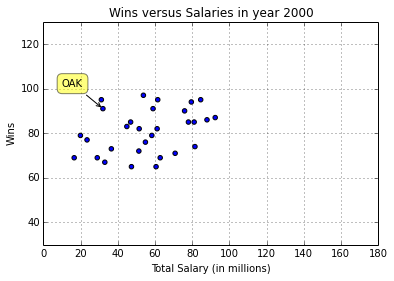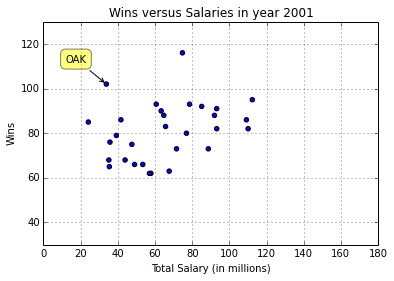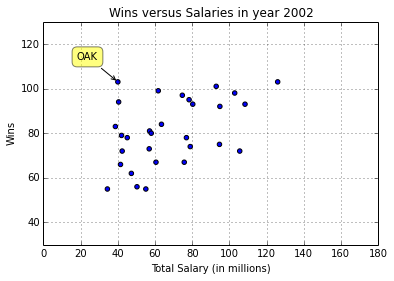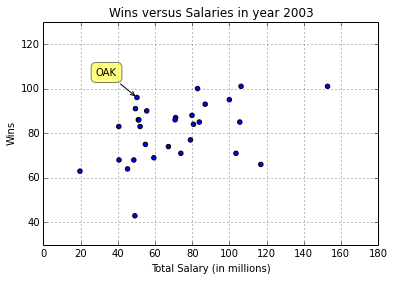We see a competitive advantage can be detected in years 2001-2003 for the Oakland baseball team, because in those years Oakland spent much less in salary compared to other teams, but stood out with the number of wins.

#### Problem 1(e):¶

For AC209 Students: Fit a linear regression to the data from each year and obtain the residuals. Plot the residuals against time to detect patterns that support your answer in 1(d).

In :
#your code here


For each year, we perform the following:

1. Calculate the least squares estimate of the coefficients in a linear regression model where x = salaries (in millions) and y = total wins.
2. Calculate the residuals for each team: $$e_i = y_i - \hat{y}_i$$
3. Plot the residuals for each team across time.
In :
teamName = 'OAK'
years = np.arange(1999, 2005)
residData = pd.DataFrame()

for yr in years:
df = joined[joined['yearID'] == yr]
x_list = df['salary'].values / 1e6
y_list = df['W'].values

# least squares estimates
A = np.array([x_list, np.ones(len(x_list))])
y = y_list
w = np.linalg.lstsq(A.T,y) # coefficients
yhat = (w*x_list+w) # regression line
residData[yr] = y - yhat

residData.index = df['teamID']
residData = residData.T
residData.index = residData.index.format()

residData.plot(title = 'Residuals from least squares estimates across years', figsize = (15, 8),
color=map(lambda x: 'blue' if x=='OAK' else 'gray',df.teamID))
plt.xlabel('Year')
plt.ylabel('Residuals')
plt.show()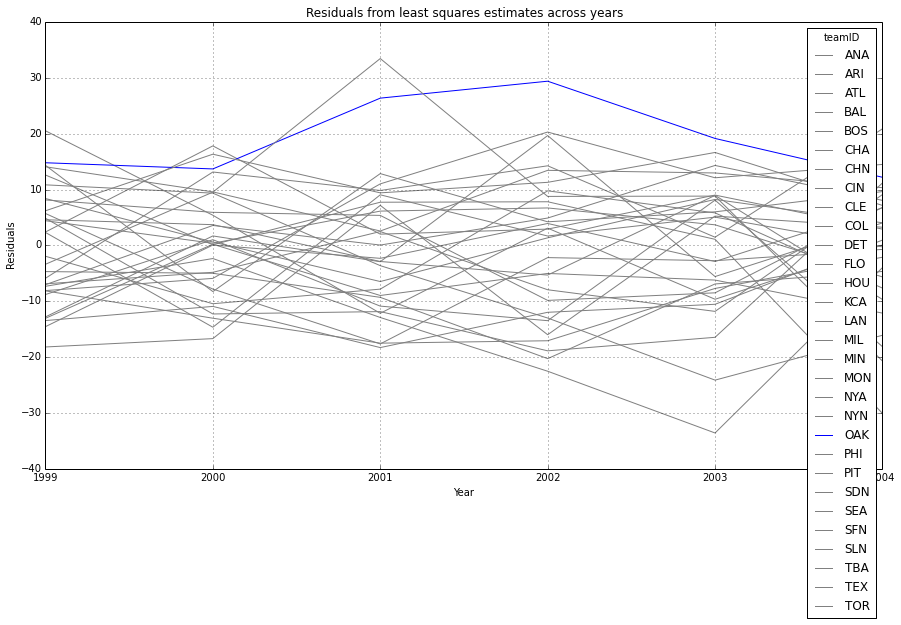## Discussion for Problem 1¶

Write a brief discussion of your conclusions to the questions and tasks above in 100 words or less.

Considering the plots from 1(d) and 1(e), we see the Oakland baseball team stood out amongst the other baseball teams in terms of their ability to win a large amount of games with a small budget from 2001-2003. Upon futher reading, we can attributed this to Billy Beane's effort to use "sabermetrics" (or the empirical analysis of baseball data) at the Oakland A's. He was able to find the most undervalued players and baseball and hire them on a reduced budget.

## Problem 2¶

Several media reports have demonstrated the income inequality has increased in the US during this last decade. Here we will look at global data. Use exploratory data analysis to determine if the gap between Africa/Latin America/Asia and Europe/NorthAmerica has increased, decreased or stayed the same during the last two decades.

#### Problem 2(a)¶

Using the list of countries by continent from World Atlas data, load in the countries.csv file into a pandas DataFrame and name this data set as countries. This data set can be found on Github in the 2014_data repository here.

In :
#your code here

url = "https://raw.githubusercontent.com/cs109/2014_data/master/countries.csv"
s = StringIO.StringIO(requests.get(url).content)
countries = pd.read_csv(s)
countries.head()

Out:
Country Region
0 Algeria AFRICA
1 Angola AFRICA
2 Benin AFRICA
3 Botswana AFRICA
4 Burkina AFRICA

Using the data available on Gapminder, load in the Income per person (GDP/capita, PPP$inflation-adjusted) as a pandas DataFrame and name this data set as income. Hint: Consider using the pandas function pandas.read_excel() to read in the .xlsx file directly. In : #your code here income_link = 'https://spreadsheets.google.com/pub?key=phAwcNAVuyj1jiMAkmq1iMg&output=xls' source = StringIO.StringIO(requests.get(income_link).content) income = pd.read_excel(source, sheetname = "Data") income.head()  Out: gdp pc test 1800 1801 1802 1803 1804 1805 1806 1807 1808 ... 2003 2004 2005 2006 2007 2008 2009 2010 2011 2012 0 Abkhazia NaN NaN NaN NaN NaN NaN NaN NaN NaN ... NaN NaN NaN NaN NaN NaN NaN NaN NaN NaN 1 Afghanistan 472.053500 472.053500 472.053500 472.053500 472.053500 472.053500 472.053500 472.053500 472.053500 ... 785.127571 804.717458 874 887.914578 983.652314 984.805841 1154.859365 1214.613653 1261.354184 1349.696941 2 Akrotiri and Dhekelia NaN NaN NaN NaN NaN NaN NaN NaN NaN ... NaN NaN NaN NaN NaN NaN NaN NaN NaN NaN 3 Albania 601.215222 601.839631 602.464688 603.090394 603.716751 604.343757 604.971415 605.599725 606.228687 ... 4855.210024 5115.252837 5369 5652.049321 5958.021197 6365.530359 6550.896164 6746.445312 6914.267317 6969.306283 4 Algeria 766.253664 766.234779 766.215895 766.197011 766.178127 766.159244 766.140362 766.121480 766.102598 ... 5576.851564 5790.967692 6011 6022.270940 6133.782763 6162.719840 6173.729741 6300.648214 6354.640523 6419.127829 5 rows × 214 columns Transform the data set to have years as the rows and countries as the columns. Show the head of this data set when it is loaded. In : #your code here income.index=income[income.columns] # Make the countries as the index income = income.drop(income.columns, axis = 1) income.columns = map(lambda x: int(x), income.columns) # Convert years from floats to ints income = income.transpose() income.head()  Out: gdp pc test Abkhazia Afghanistan Akrotiri and Dhekelia Albania Algeria American Samoa Andorra Angola Anguilla Antigua and Barbuda ... Western Sahara Vietnam Virgin Islands (U.S.) Yemen Arab Republic (Former) Yemen Democratic (Former) Yemen, Rep. Yugoslavia Zambia Zimbabwe Åland 1800 NaN 472.0535 NaN 601.215222 766.253664 674.453726 1260.123256 359.932582 775.668711 538.376199 ... NaN 459.708986 NaN NaN NaN 661.902376 NaN 364.464811 372.818338 NaN 1801 NaN 472.0535 NaN 601.839631 766.234779 674.453726 1262.214402 359.932582 775.668711 538.376199 ... NaN 459.708986 NaN NaN NaN 662.058563 NaN 364.464811 372.818338 NaN 1802 NaN 472.0535 NaN 602.464688 766.215895 674.453726 1264.309018 359.932582 775.668711 538.376199 ... NaN 459.708986 NaN NaN NaN 662.214787 NaN 364.464811 372.818338 NaN 1803 NaN 472.0535 NaN 603.090394 766.197011 674.453726 1266.407109 359.932582 775.668711 538.376199 ... NaN 459.708986 NaN NaN NaN 662.371047 NaN 364.464811 372.818338 NaN 1804 NaN 472.0535 NaN 603.716751 766.178127 674.453726 1268.508683 359.932582 775.668711 538.376199 ... NaN 459.708986 NaN NaN NaN 662.527345 NaN 364.464811 372.818338 NaN 5 rows × 260 columns #### Problem 2(b)¶ Graphically display the distribution of income per person across all countries in the world for any given year (e.g. 2000). What kind of plot would be best? In : #your code here  Here we use a histogram to plot the distribution of income per person in a given year across all the countries on the dollar scale and the log10(dollar) scale. In : year = 2000 plt.plot(subplots=True) plt.hist(income.ix[year].dropna().values, bins = 20) plt.title('Year: %i' % year) plt.xlabel('Income per person') plt.ylabel('Frequency') plt.show() plt.hist(np.log10(income.ix[year].dropna().values), bins = 20) plt.title('Year: %i' % year) plt.xlabel('Income per person (log10 scale)') plt.ylabel('Frequency') plt.show()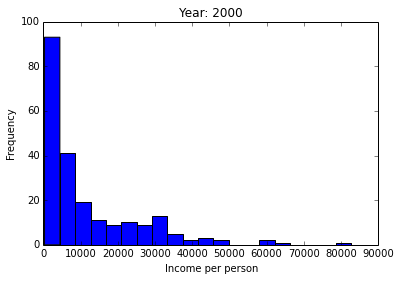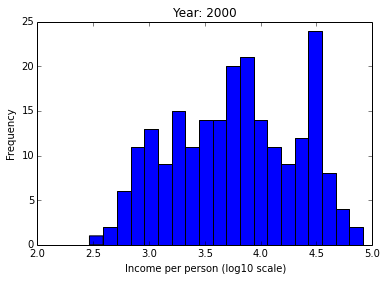#### Problem 2(c)¶ Write a function to merge the countries and income data sets for any given year. In : """ Function -------- mergeByYear Return a merged DataFrame containing the income, country name and region for a given year. Parameters ---------- year : int The year of interest Returns ------- a DataFrame A pandas DataFrame with three columns titled 'Country', 'Region', and 'Income'. Example ------- >>> mergeByYear(2010) """ #your code here def mergeByYear(year): data = pd.DataFrame(income.ix[year].values, columns = ['Income']) data['Country'] = income.columns joined = pd.merge(data, countries, how="inner", on=['Country']) joined.Income = np.round(joined.Income, 2) return joined mergeByYear(2010).head()  Out: Income Country Region 0 1214.61 Afghanistan ASIA 1 6746.45 Albania EUROPE 2 6300.65 Algeria AFRICA 3 33052.28 Andorra EUROPE 4 5497.62 Angola AFRICA #### Problem 2(d)¶ Use exploratory data analysis tools such as histograms and boxplots to explore the distribution of the income per person by region data set from 2(c) for a given year. Describe how these change through the recent years? Hint: Use a for loop to consider multiple years. In : #your code here years = np.arange(1950, 2010, 10) for yr in years: df = mergeByYear(yr) df.boxplot('Income', by = 'Region', rot = 90) plt.title("Year:" + str(yr)) plt.ylabel('Income per person (log10 scale)') plt.ylim(10**2, 10.5 **5) plt.yscale('log')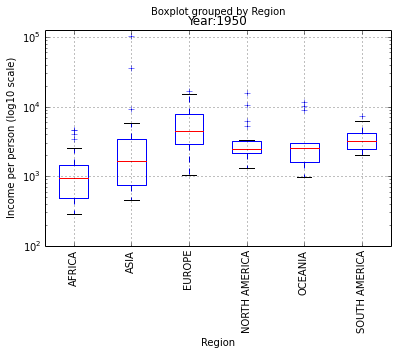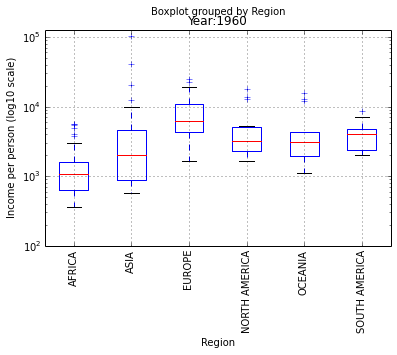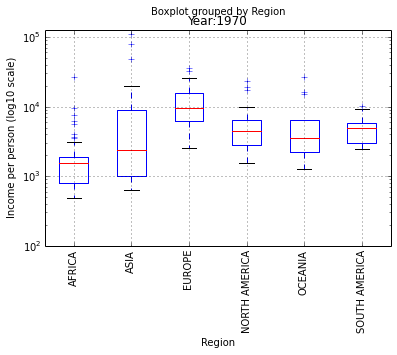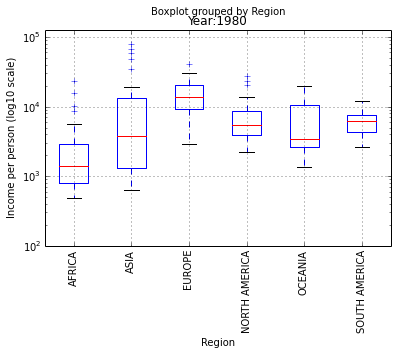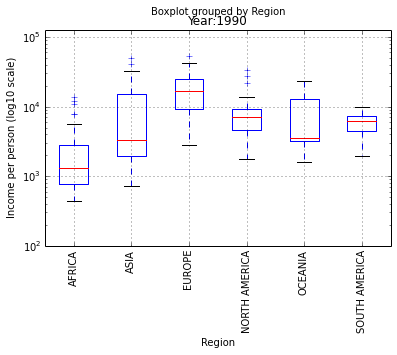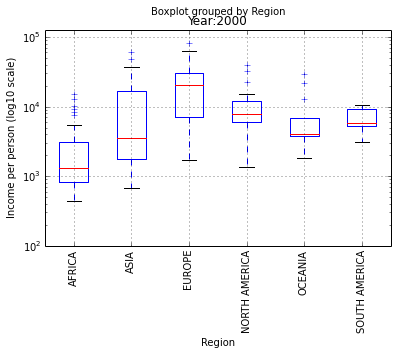In recent years, Africa and Asia have an upwards trend in average income per person while other continents have stayed more constant through the 20th century. ## Discussion for Problem 2¶ Write a brief discussion of your conclusions to the questions and tasks above in 100 words or less. In most continents (especially Africa and Asia), we see that the distribution of incomes is very skewed: most countries are in a group of low-income states with a fat tail of high-income countries that remains approximately constant throughout the 20th century. In 2(b) we used a histogram to look at the income distribution of all countries in the world for a given year saw a non-normal distribution. In 2(d) we used boxplots to take a closer look at the income distribution of countries grouped by regions across decades. We saw upward trends in average income per person across certain regions (e.g. Africa) as we moved through the 20th century. ## Problem 3¶ In general, if group A has larger values than group B on average, does this mean the largest values are from group A? Discuss after completing each of the problems below. #### Problem 3(a)¶ Assume you have two list of numbers, X and Y, with distribution approximately normal. X and Y have standard deviation equal to 1, but the average of X is different from the average of Y. If the difference in the average of X and the average of Y is larger than 0, how does the proportion of X > a compare to the proportion of Y > a? Write a function that analytically calculates the ratio of these two proportions: Pr(X > a)/Pr(Y > a) as function of the difference in the average of X and the average of Y. Hint: Use the scipy.stats module for useful functions related to a normal random variable such as the probability density function, cumulative distribution function and survival function. Update: Assume Y is normally distributed with mean equal to 0. Show the curve for different values of a (a = 2,3,4 and 5). In : """ Function -------- ratioNormals Return ratio of these two proportions: Pr(X > a)/Pr(Y > a) as function of the difference in the average of X and the average of Y. Parameters ---------- diff : difference in the average of X and the average of Y. a : cutoff value Returns ------- Returns ratio of these two proportions: Pr(X > a)/Pr(Y > a) Example ------- >>> ratioNormals(diff = 1, a = 2) """ #your code here def ratioNormals(diff, a): X = scipy.stats.norm(loc=diff, scale=1) Y = scipy.stats.norm(loc=0, scale=1) return X.sf(a) / Y.sf(a)  In : #your code here # let diff range from 0 to 5 diffs = np.linspace(0, 5, 50) a_values = range(2,6) # Plot separate curves for # Pr(X > a) / Pr(Y > a) as a function of diff # for all given values of a plt.figure(figsize=(8,5)); for a in a_values: ratios = [ratioNormals(diff, a) for diff in diffs] plt.plot(diffs, ratios) # Labels plt.legend(["a={}".format(a) for a in a_values], loc=0); plt.xlabel('Diff'); plt.ylabel('Pr(X>a) / Pr(Y>a)'); plt.title('Ratio of Pr(X > a) to Pr(Y > a) as a Function of Diff'); # Using a log scale so you can actually see the curves plt.yscale('log')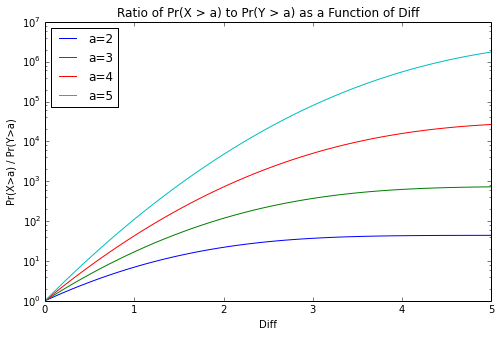#### Problem 3(b)¶ Now consider the distribution of income per person from two regions: Asia and South America. Estimate the average income per person across the countries in those two regions. Which region has the larger average of income per person across the countries in that region? Update: Use the year 2012. In : #your code here merged = mergeByYear(2012).groupby('Region', as_index=False).mean() merged = merged.loc[(merged.Region == "ASIA") | (merged.Region == "SOUTH AMERICA")] merged.Income = np.round(merged.Income, 2) merged  Out: Region Income 1 ASIA 14510.04 5 SOUTH AMERICA 9471.47 Asia has a larger income compared to South America. We can also create boxplots to see the income distribution of the two continents on the dollar scale and log10(dollar) scale. In : df = mergeByYear(2012) df = df.loc[(df.Region == "ASIA") | (df.Region == "SOUTH AMERICA")] df.boxplot('Income', by = 'Region', rot = 90) plt.ylabel('Income per person (dollars)')  Out: <matplotlib.text.Text at 0x10b09ba90>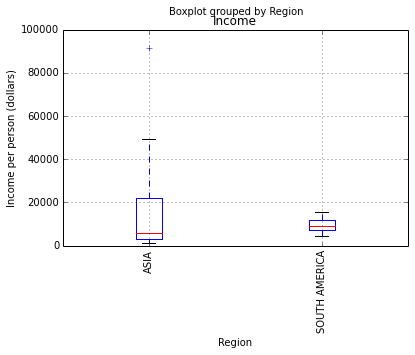In : df = mergeByYear(2012) df = df.loc[(df.Region == "ASIA") | (df.Region == "SOUTH AMERICA")] df.boxplot('Income', by = 'Region', rot = 90) plt.ylabel('Income per person (log10 scale)') plt.yscale('log')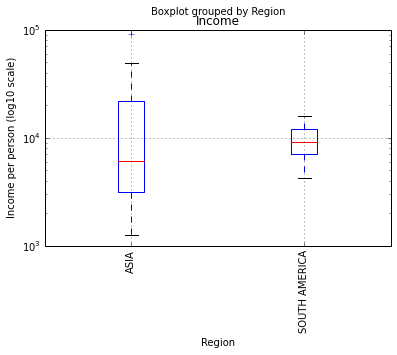#### Problem 3(c)¶ Calculate the proportion of countries with income per person that is greater than 10,000 dollars. Which region has a larger proportion of countries with income per person greater than 10,000 dollars? If the answer here is different from the answer in 3(b), explain why in light of your answer to 3(a). Update: Use the year 2012. In : def ratioCountries(groupedData, a): prop = [len(group.Income[group.Income >= a]) / float(len(group.Income.dropna())) for key, group in groupedData] z = pd.DataFrame(groupedData.mean().index, columns = ['Region']) z['Mean'] = np.round(groupedData.mean().values,2) z['P(X > %g)' % a] = np.round(prop, 4) return z df = mergeByYear(2012).groupby('Region') df_ratio = ratioCountries(df, 1e4) df_ratio = df_ratio[(df_ratio.Region == 'ASIA') | (df_ratio.Region == 'SOUTH AMERICA')] df_ratio  Out: Region Mean P(X > 10000) 1 ASIA 14510.04 0.3684 5 SOUTH AMERICA 9471.47 0.4167 South America has a larger proportion of countries greater than 10,000 dollars compared to Asia. This is different from the answer in 3(b). Because Asia does not have a normal distribution, the theory in Problem in 3(a) is not applicable here. #### Problem 3(d)¶ For AC209 Students: Re-run this analysis in Problem 3 but compute the average income per person for each region, instead of the average of the reported incomes per person across countries in the region. Why are these two different? Hint: use this data set. In : #your code here # First import the population data: population_link = 'https://spreadsheets.google.com/pub?key=phAwcNAVuyj0XOoBL_n5tAQ&output=xls' source = StringIO.StringIO(requests.get(population_link).content) population = pd.read_excel(source, sheetname = "Data") # Put years as index and countries as column names population.columns = ['Country'] + map(int, list(population.columns)[1:]) population.head()  Out: Country 1700 1730 1750 1785 1786 1787 1788 1789 1790 ... 2004 2005 2006 2007 2008 2009 2010 2011 2012 2013 0 Abkhazia NaN NaN NaN NaN NaN NaN NaN NaN NaN ... NaN NaN NaN NaN NaN NaN NaN NaN NaN NaN 1 Afghanistan NaN NaN NaN NaN NaN NaN NaN NaN NaN ... 26693486 27614718 28420974 29145841 29839994 30577756 31411743 32358260 33397058 34499915 2 Akrotiri and Dhekelia NaN NaN NaN NaN NaN NaN NaN NaN NaN ... NaN 15700 NaN NaN 15700 NaN NaN NaN NaN NaN 3 Albania 300000 NaN NaN NaN NaN NaN NaN NaN NaN ... 3124861 3141800 3156607 3169665 3181397 3192723 3204284 3215988 3227373 3238316 4 Algeria 1750000 NaN NaN NaN NaN NaN NaN NaN NaN ... 32396048 32888449 33391954 33906605 34428028 34950168 35468208 35980193 36485828 36983924 5 rows × 233 columns Consider we have$M$regions. Let$R_j$be the number of countries in the$j^{th}$region where$j = 1, \ldots, M$. If the income per person in a country$i$is$I_i$, we previously calculated the average income per person over$R_j$countries in the$j^{th}$region using the following formula: $$\bar{S}_j = \frac{1}{R_j}\sum_{i=1}^{R_j} I_i$$ Now, we want to consider the average income in a given region adjusting for each countries' population$N_i$. Therefore, we use the following formula to calculate an average income in a given region$R_j\$ adjusting for the population in each country: $$\bar{S}_j = \frac{S_1 + S_2 + \ldots + S_{R_j}}{N_1 + N_2 + \ldots + N_{R_j} } = \frac{N_1 * I_1 + N_2 * I_2 + \ldots + N_{R_j} * I_{R_j}}{N_1 + N_2 + \ldots + N_{R_j} }$$

We start by creating a function similar to mergeByYear() from 2(c) which merges the income for each country in a given region, but also includes the population for each country.

In :
def mergeByYearWithPop(year):

# income DataFrame
income_df = pd.DataFrame(income.ix[year].values, columns = ['Income'])
income_df['Country'] = income.columns

# merge income DataFrame and countries
joined = pd.merge(income_df, countries, how="inner", on=['Country'])

# population DataFrame
population_df = population[['Country',year]]

# merge population DataFrame and joined DataFrame
joined = pd.merge(joined, population_df, how="inner", on=['Country'])
joined.columns = list(joined.columns[:-1])+['TotalPopulation']
joined.Income = np.round(joined.Income, 2)

def func(df):
totPop = df.sum()['TotalPopulation']
dfout = df
dfout['AdjustedIncome'] = df.Income * df.TotalPopulation / float(totPop)
dfout.AdjustedIncome = np.round(dfout.AdjustedIncome, 2)
return dfout

# Group by region
returnDataFrame = joined.groupby('Region').apply(func)

return returnDataFrame

mergeByYearWithPop(2012).head()

Out:
Income Country Region TotalPopulation AdjustedIncome
0 1349.70 Afghanistan ASIA 33397058 11.13
1 6969.31 Albania EUROPE 3227373 37.68
2 6419.13 Algeria AFRICA 36485828 246.02
3 NaN Andorra EUROPE 87518 NaN
4 5838.16 Angola AFRICA 20162517 123.65
In :
df = mergeByYearWithPop(2012).groupby('Region').sum()
df.Income = mergeByYear(2012).groupby('Region').mean().Income
df.Income = np.round(df.Income, 2)
df = df.ix[['ASIA', 'SOUTH AMERICA']]
df

Out:
Income TotalPopulation AdjustedIncome
ASIA 14510.04 4048448110 6731.66
SOUTH AMERICA 9471.47 400557572 10550.87

In 3(b) we computed the average income per person in each of the regions. The first column in the table above contains the averages from 3(b) (i.e. without adjusting for population). Column 2 contains the total population in the given region. The last column is the average income per person in each of the regions (adjusted for population).

To compare the boxplots from 3(b), here we plot the income per person for a given region (adjusted for population) on the log10 scale.

In :
df = mergeByYearWithPop(2012)
df.AdjustedIncome = df['AdjustedIncome']
df = df[(df.Region == 'ASIA') | (df.Region == 'SOUTH AMERICA')]
df.boxplot('AdjustedIncome', by = 'Region', rot = 90)
plt.yscale('log')
plt.ylabel('Income per person adjusted for population (log10 scale)')

Out:
<matplotlib.text.Text at 0x10ba6d250>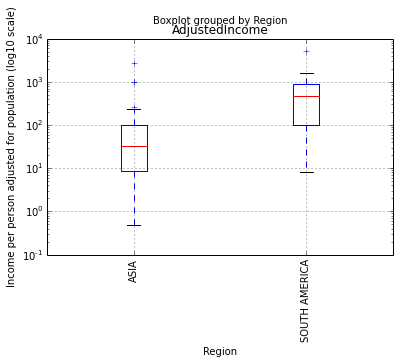Next let's repeat the analysis in 3(c).

In :
def ratioCountries(groupedData, a):
prop = [len(group.AdjustedIncome[group.AdjustedIncome >= a]) / float(len(group.AdjustedIncome.dropna())) for key, group in groupedData]
z = pd.DataFrame(groupedData.mean().index, columns = ['Region'])
z['AdjustedIncome'] = np.round(groupedData.AdjustedIncome.sum().values,2)
z['P(X > %g)' % a] = np.round(prop,4)
return z

In :
df = mergeByYearWithPop(2012).groupby('Region')
df_ratio = ratioCountries(df, 1e4)
df_ratio = df_ratio[(df_ratio.Region == 'ASIA') | (df_ratio.Region == 'SOUTH AMERICA')]
df_ratio

Out:
Region AdjustedIncome P(X > 10000)
1 ASIA 6731.66 0
5 SOUTH AMERICA 10550.87 0

If we lower the value a from 10,000 to 1,000:

In :
df = mergeByYearWithPop(2012).groupby('Region')
df_ratio = ratioCountries(df, 1e3)
df_ratio = df_ratio[(df_ratio.Region == 'ASIA') | (df_ratio.Region == 'SOUTH AMERICA')]
df_ratio

Out:
Region AdjustedIncome P(X > 1000)
1 ASIA 6731.66 0.0526
5 SOUTH AMERICA 10550.87 0.1667

The solutions to Problem 3(d) are different because here we are correcting for the countries with the largest populations.

## Discussion for Problem 3¶

Write a brief discussion of your conclusions to the questions and tasks above in 100 words or less.

The start of Problem 3 asked: If group A has larger values than group B on average, does this mean the largest values are from group A?. After completely Problem 3, we see the answer is no. In 2012, Asia had a larger average income per person compared to South America, but because the distributions of Asia and South America are different (e.g. Asia is not normally distributed), we saw the probability of seeing extreme values in Asia is smaller than the probability of seeing extreme values in South America. When we adjust the incomes for the population of each country, we are correcting for the problem of outliers or the countries with the largest populations.

In :